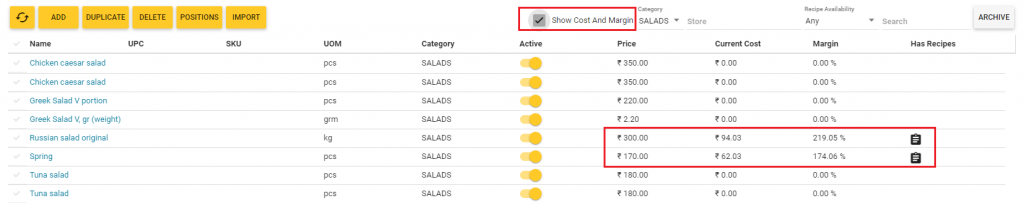## Overview

This topic explains how to view and control the cost and margin for menu items with active recipes

A recipe specifies a list of ingredients of a base menu item, and the average cost and weight of each ingredient per a portion and a unit of measurement of the base item.  The sum of costs of each ingredient is referred to as the cost of materials. Optionally, the recipe specifies a fixed cost per a unit of the base item.

TIP: Net cost (or cost) of a menu item unit is calculated as a sum of the cost of materials and a fixed cost, if any. For more information and examples, see “Net Cost of Menu Items with Active Recipes” in How to Use Recipes.

To find a margin, it is necessary to divide the menu item price by the item cost. For example, if a salad portion price is 200 (in the currency units), and a salad portion cost is 100, the margin for this menu item is calculated as following:

Margin = 200/100 x 100 % = 200%

NOTE: YumaPOS calculates the cost and margin only for menu items with active recipes.

## Display the Current Cost and Margin

To display the current values of cost and margin for menu items, perform the following steps:

1. In the left pane of Back Office Management console, expand the Items List node, and then click Items List.
2. Select the Show Cost and Margin check box.
This displays a list of menu items with their costs and margin similar to the following screen:IMPORTANT: The Current Cost and Margin columns display the items costs and margin only for items with active recipes. For other items, these values are always set to zero.

TIP: You can filter the menu items list by categories and stores where the items are available.

## How YumaPOS Calculates a Menu Item Cost

Please note that a menu item cost is not fixed: it depends on the current costs of ingredients (materials) included in the menu item recipe. YumaPOS calculates the menu item cost automatically.

To illustrate a procedure for calculating the average cost of materials for preparing a salad, consider the following example.

A salad recipe includes tomatoes. There are two lots of tomatoes in a location:

• Lot1: 20 kg (the purchase price is 25 currency units (c.u.)/kg)
• Lot2: 30 kg (the purchase price is 40 c.u./kg).

In this case, the average cost of tomatoes is calculated using the following expression:

Av. Cost=  (20  x 25  + 30  x 40) / (20 +30) = 1700 /50 = 34 (c.u./kg)

However, if you received another lot of tomatoes, such as Lot3: 50 kg  (the purchase price is 36 c.u./kg), the current cost changes. Now it is calculated in the following way:

Av. Cost = (20 x 25 + 30 x 40 + 50 x 36)/ (20 + 30 +50) = 3500 / 100 = 35 (c.u./kg)

NOTE: Selecting the Show Cost and Margin check box on the Items list page (see the screen earlier in this document) updates the current costs and margin of all menu items with active recipes.

### Operations That Change the Cost

The following operations may change a menu item cost (the list is not complete):

• Receiving the menu item ingredients (for more information, see Acts of Receiving): this operation increases the ingredients quantity and changes the average cost.
• Writing-off the menu item ingredients (for more information, see Write-off Acts): this operation decreases the ingredients quantity.
• Selling menu items with active recipes: this operation decreases the ingredients quantity.

## How to Optimize a Menu Item Margin

An optimal value of a menu item margin depends on your price policy and specific features of your business. As a rule, an optimal margin is about 200%.

If for some menu items the margin takes a low value (such as 100-120%), the first step is to figure out why. Depending on your situation, consider the following ways to increase the margin:

• Increase the menu item price (note that this may result in sales dip).
• Try to lower the item ingredients cost (for example, to buy ingredients, try to find other contractors that offer lower purchase prices).
• Try to lower the fixed costs applied to the menu item recipe.